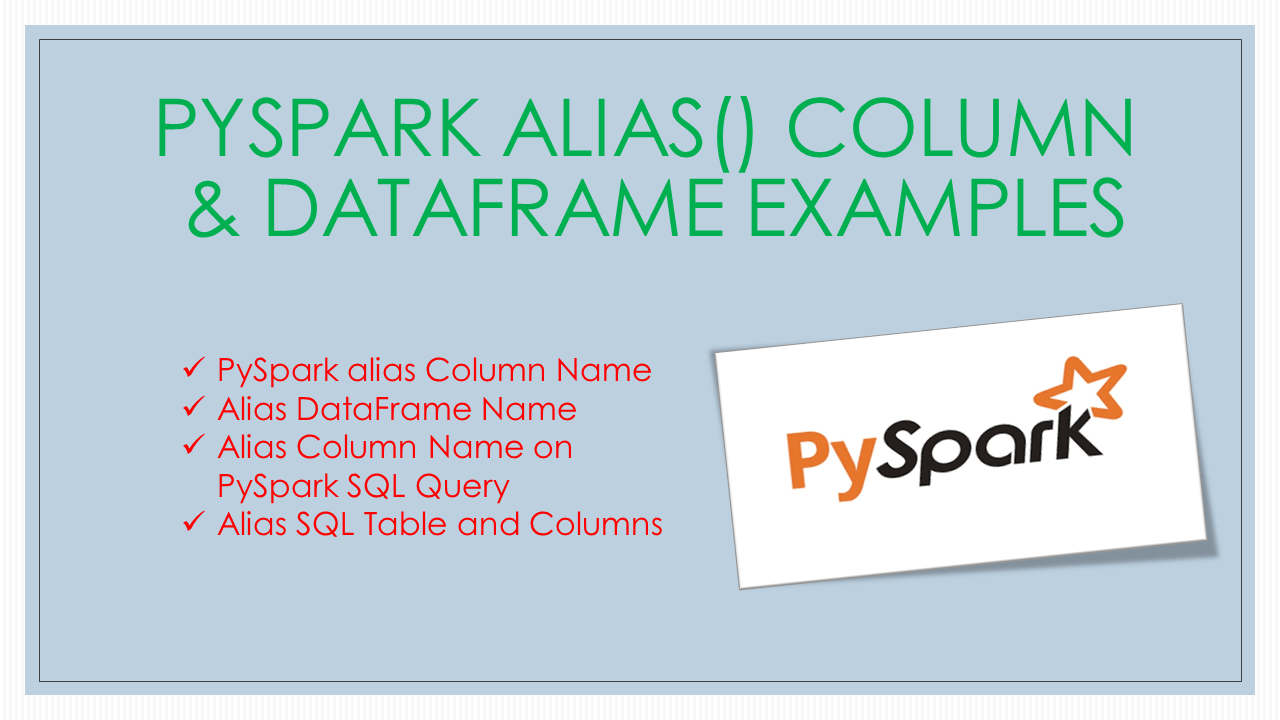# PySpark alias() Column & DataFrame Examples

How to create an alias in PySpark for a column, DataFrame, and SQL Table? We are often required to create aliases for several reasons, one of them would be to specify user understandable names for coded names. For example, if you have `fname`, you may want to use `first_name`.

Alias of PySpark DataFrame column changes the name of the column without changing the type and the data. DataFrame and SQL table alias give a different name to the DataFrame/table without changing the structure, data, and column names.

## 1. Quick Examples of PySpark Alias

Below are some of the quick examples of how to alias column name, DataFrame, and SQL table in PySpark.

``````
# Example 1 - Column.alias()
df.select("fee",df.lang.alias("language")).show()

# Example 2 - using col().alias() - col() return Column type
from pyspark.sql.functions import col
df.select("fee",col("lang").alias("language")).show()

# Example 3 - Query using spark.sql() and use 'as' for alias
spark.sql("select fee, lang as language from courses").show()

# Example 4 - Query using spark.sql() and use 'as' for alias
spark.sql("select subject.fee, subject.lang as language from courses as subject").show()
``````

Let’s create a DataFrame

``````
# Import
from pyspark.sql import SparkSession

# Create SparkSession
spark = SparkSession.builder.master("local") \
.appName('SparkByExamples.com') \
.getOrCreate()

# Create DataFrame
columns = ["lang","fee"]
data = [("Java", 20000), ("Python", 10000), ("Scala", 10000)]
df = spark.createDataFrame(data).toDF(*columns)
``````

## 2. PySpark alias Column Name

`pyspark.sql.Column.alias()` returns the aliased with a new name or names. This method is the SQL equivalent of the `as` keyword used to provide a different column name on the SQL result.

Following is the syntax of the Column.alias() method.

``````
# Syntax of Column.alias()
Column.alias(*alias, **kwargs)
``````

Parameters

• `alias` – column name you wanted to alias to.
• `kwargs` – kwargs values.

Now, let’s run an example with a column alias. Since DataFrame is immutable, this returns a new DataFrame with an alias column name. You can use this to alias one or multiple columns at a time.

``````
# Alias column name
df2 = df.select("fee",df.lang.alias("language"))
df2.printSchema()

# Using col() function
from pyspark.sql.functions import col
df.select("fee",col("lang").alias("language")).show()
``````

Above both examples yields the below output. Notice the column change from `lang` to `language` with alias.

``````
# Output
+-----+--------+
|  fee|language|
+-----+--------+
|20000|    Java|
|10000|  Python|
|10000|   Scala|
+-----+--------+

root
|-- fee: long (nullable = true)
|-- language: string (nullable = true)
``````

## 3. Alias DataFrame Name

You can also alias the DataFrame name. If you are not aware you can set the name to the DataFrame that shows on DAG on PySpark web UI. You can change this name by using DataFrame.alias()

``````
# Alias DataFrmae name
df.alias('df_one')
``````

## 4. Alias Column Name on PySpark SQL Query

If you have some SQL background you would know that `as` is used to provide an alias name of the column, similarly even in PySpark SQL, you can use the same notation to provide aliases.

Let’s see with an example. In order to use SQL query, first, you need to create a temporary view of the DataFrame so that you can run SQL query. A temporary view can be created using `DataFrame.createOrReplaceTempView()`

``````
# Create SQL table
df.createOrReplaceTempView("Courses")

# Query using spark.sql() and use 'as' for alias
spark.sql("select fee, lang as language from courses").show()
``````

Yields same DataFrame output as above. Note that the scope of the `courses` table is with the PySpark Session. Once the session closed you can’t access this table

## 5. Alias SQL Table and Columns

Now let’s alias the name of the table in SQL and the column name at the same time. Alias of column names would be very useful when you are working with joins.

``````
# Query using spark.sql() and use 'as' for alias
df4 = spark.sql("select subject.fee, subject.lang as language from courses as subject")
df4.show()
``````

## 6. Conclusion

In this article, you have learned how to alias column names using an alias(). This method is the SQL equivalent of the keyword used to provide a different column name on the SQL result. Also, learned how to use an alias on SQL queries after creating a table from DataFrame.

### Naveen (NNK)

Naveen (NNK) is a Data Engineer with 20+ years of experience in transforming data into actionable insights. Over the years, He has honed his expertise in designing, implementing, and maintaining data pipelines with frameworks like Apache Spark, PySpark, Pandas, R, Hive and Machine Learning. Naveen journey in the field of data engineering has been a continuous learning, innovation, and a strong commitment to data integrity. In this blog, he shares his experiences with the data as he come across. Follow Naveen @ @ LinkedIn